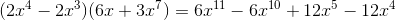Example Questions

2 Next →

Example Question #81 : Exponents

Distribute and simplify: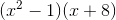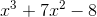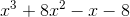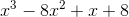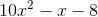Explanation:

To FOIL this binomial distribution, we simply distribute the terms in a specific order:Multiply the First terms: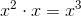Multiply the Outer terms: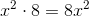Multiply the Inner terms: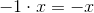Multiply the Last terms: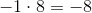Lastly, combine any terms that allow this (usually, but not always, the two middle terms). In this case, no two terms are compatible.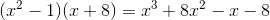Example Question #82 : Exponents

Distribute and simplify: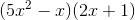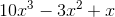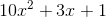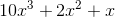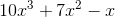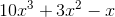Explanation:

To FOIL this binomial distribution, we simply distribute the terms in a specific order:Multiply the First terms: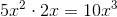Multiply the Outer terms: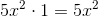Multiply the Inner terms: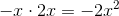Multiply the Last terms: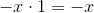Lastly, combine any terms that allow this (usually, but not always, the two middle terms).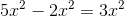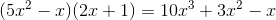Example Question #83 : Exponents

Distribute and simplify: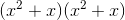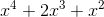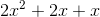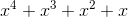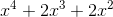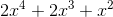Explanation:

To FOIL this binomial distribution, we simply distribute the terms in a specific order:Multiply the First terms: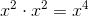Multiply the Outer terms:Multiply the Inner terms: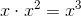Multiply the Last terms: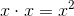Lastly, combine any terms that allow this (usually, but not always, the two middle terms).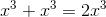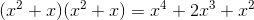Example Question #84 : Exponents

Distribute and simplify: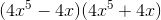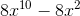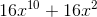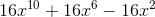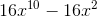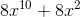Explanation:

A clever eye might spot that this binomial takes the form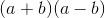, which means you can jump straight to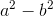as the answer. But let's look at it in detail below.

To FOIL this binomial distribution, we simply distribute the terms in a specific order:Multiply the First terms: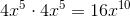Multiply the Outer terms: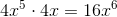Multiply the Inner terms: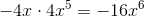Multiply the Last terms: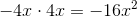Lastly, combine any terms that allow this (usually, but not always, the two middle terms).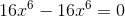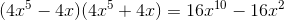Example Question #85 : Exponents

Distribute and simplify: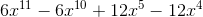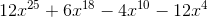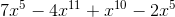Explanation:

To FOIL this binomial distribution, we simply distribute the terms in a specific order: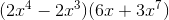Multiply the First terms: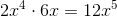Multiply the Outer terms: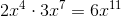Multiply the Inner terms: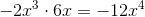Multiply the Last terms: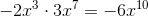Lastly, combine any terms that allow this (usually, but not always, the two middle terms). In this case, no two terms are compatible.